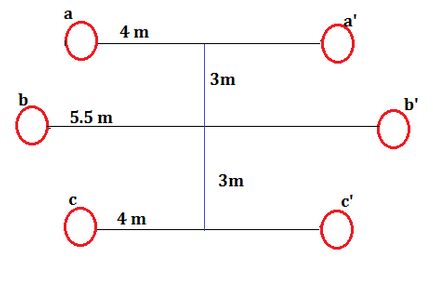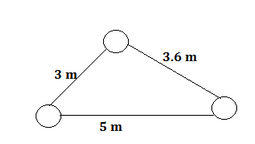# Line Inductance of a three phase circuit - MCQs with Answers

## Line Inductance of a three phase circuit - MCQs with Answers

Q1. Why is the transmission lines transposed?

a. Reduce corona loss.
b. Reduce skin effect.
c. Prevent interference with the neighbouring telephone lines.
d. Prevent short circuit between any two lines.

ANSWER: c. Prevent interference with the neighbouring telephone lines.

Q2. A three phase, 50Hz transmission line consists of three equal conductors of radii ‘r’ placed in a horizontal plane with a spacing of 6m between middle and outer conductor. If the radius of each conductor is 12.5 mm, then inductance per phase per km of the transposed line will be

a. 1.33 mH/km
b. 1.65 mH/km
c. 0.38 mH/km
d. 2.145 mH/km

ANSWER: a. 1.33 mH/km

Q3. A three phase transmission line has its conductors at the corners of an equilateral triangle with sides 3m. The diameter of each conductor is 1.63 cm. What is the inductance of the line per phase?

a. 1.232 mH
b. 1.184 mH
c. 2.236 mH
d. 2.68 mH

ANSWER: a. 1.232 mH

Q4. What will be the inductance of each conductor in a three phase, 3 wire system when they are arranged in a horizontal plane with spacing such that D31= 4m, D12 = D23 = 2m. The conductors are transposed and have a diameter of 2.5 cm.

a. 2.22 mH
b. 1.11 mH
c. 3.33 mH
d. 4.44 mH

ANSWER: b. 1.11 mH

Q5. What is the inductance per phase per km of a double circuit 3 phase line as shown in the fig. The conductors are transposed and are of radius 0.75 cm each.a. 0.725 mH
b. 0.835 mH
c. 0.623 mH
d. 0.231 mH

ANSWER: c. 0.623 mH

Q6. Why is high voltage transmission lines transposed?

a. Corona losses can be minimised.
b. Computation of inductance becomes easier.
c. Voltage drops in the lines can be minimised.
d. Phase voltage imbalances can be minimised.

ANSWER: d. Phase voltage imbalances can be minimised.

Q7. The three phase line consists of three conductors each of diameter 21 mm. The spacing is shown in fig. Find the inductance per phase/ km.a. 8.2 * 10-4 H / km
b. 12.22 * 10-4 H / km
c. 5.6 * 10-4 H / km
d. None of these.

ANSWER: b. 12.22 * 10-4 H / km

Q8. When is the transposition of conductors in a transmission line done?

a. When the conductors are not equally spaced.
b. When the conductors are spaced equilaterally.
c. When a telephone line runs parallel to the power line.
d. None of these.

ANSWER: a. When the conductors are not equally spaced.

Q9. Assertion (A): Transposition of conductors in a transmission line is necessary.
Reason (R): Corona losses are reduced by transposition of conductors.

a. Both A and R are true and R is the correct explanation of A
b. Both A and R are true and R is not the explanation of A
c. A is true but R is false
d. A is false but R is true.

ANSWER: c. A is true but R is false

Q10. Assertion (A): the concept of GMD is applicable for the calculation of inductance of transmission lines.
Reason (R): The radius of the conductor of transmission lines, in the expression for inductance is not the self GMD of the conductor.

a. Both A and R are true and R is the correct explanation of A
b. Both A and R are true and R is not the explanation of A
c. A is true but R is false
d. A is false but R is true.

ANSWER: b. Both A and R are true and R is not the explanation of A

Q11. In case of stranded conductors, what is the ratio of the GMR to the actual radius?

a. Equal to 1
b. More than 1
c. Equal to 0.7788
d. Less than 0.7788

ANSWER: d. Less than 0.7788

Q12. Ds is the GMR of each subconductor of a four subconductor bundled conductor and d is the bundle spacing. What is the GMR of the equivalent single conductor?

a. 1.09 √ (Ds * d3)
b. 1.09 √ (Ds3 * d3)
c. 1.09 (√ (Ds 3 * d3))1/4
d. 1.09 (√(Ds * d3))1/4